Definitions

# Electrodynamics

Electrodynamics is the part of physics that studies the dynamic aspect of electricity, that is, the constant movement of electric charges.

## Important concepts of Electrodynamics

### → Conductors and insulators

There are materials that are capable of conducting electrical charges with relative ease. These materials are called conductors. On the other hand, there are materials that oppose the conduction of electric current, they are called insulators or dielectrics . To learn more, read: Conductors and Insulators .

### → Electric current

The electric current , which is the movement of charges, is calculated by the amount of charges that cross the cross section of a conductor every second. The unit of measurement of electric current in the International System (SI) is the ampere. Its formula is as follows: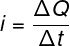i – electric current (A)

ΔQ – electric charge (C)

Δt – time interval (s)

In conductive materials, electric current is produced by the movement of electrons. These particles are endowed with a negative electrical charge of magnitude equal to 1.6.10 -19 C , known as the fundamental electrical charge . Since the electric current is formed by electrons, it can be written as follows: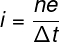n – number of electrons

e – fundamental electric charge (1,6.10 -19 C)

Don’t stop now… There’s more after the publicity 😉

### → Electrical resistance

The intensity of the electric current formed depends on a characteristic of the body called electrical resistance Bodies whose electrical resistance is constant, regardless of which voltage is applied to it, are called ohmic. These bodies obey Ohm’s 1st law , described by the following formula:U – electrical voltage (V)

R – electrical resistance (Ω)

Electrical resistance depends on geometric factors such as the length and cross-sectional area of ​​the conductor. This is explained mathematically through Ohm’s 2nd law :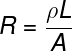L – body length (m)

A – cross-sectional area (m²)

ρ – resistivity ( Ω.m )

### → Resistivity

Resistivity, denoted by the symbol ρ , is a microscopic property of the material itself. The greater the resistivity , the greater the electrical resistance of the body. Thus, conductive materials have low resistivity.

The formula used to calculate the resistivity involves microscopic quantities and advanced knowledge of electromagnetic theory, so in high school, we don’t usually calculate it, but only use its module, when informed by the exercises.

### → Joule effect

When electric current runs through an insulating material, we say that a dielectric breakdown has occurred . When an insulating material starts to conduct electricity, a large amount of heat is produced, due to the difficulty that the electric current finds when passing between the atoms of the material. This phenomenon of heat production , known as the Joule Effect , is widely exploited in heaters, showers, crockpots , etc. The Joule Effect formula allows us to calculate the amount of electrical energy that is dissipated into thermal energy. To do so, we relate quantities such as resistance, electric current and time interval. Watch: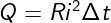Q – amount of energy transformed into heat (J)

R – electrical resistance

### → Electric power

If we wish to calculate the amount of energy converted into heat due to the Joule effect , we must determine the electrical power dissipated. Power is a quantity that measures the rate of energy transfer. Its unit of measurement is the watt , which is equivalent to joules per second.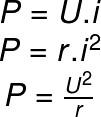P – electrical power (W)

r – electrical resistance (Ω)

## Electrodynamics in Enem

Electrodynamics is the second most popular subject among Enem questions , so when preparing for the exam, pay special attention to topics such as:

• Ohm’s laws – 1st Ohm’s law and 2nd Ohm’s law ;
• association of resistors ;
• electric generators ;
• electrical power .

In addition, try to understand the theory behind the content well, as it is as important for the test as the resolution of exercises itself. Shall we check an Enem question that involved this content?

(Enem) Every car has a fuse box, which are used to protect electrical circuits. Fuses are made of a material with a low melting point, such as tin, for example, and they melt when an electric current equal to or greater than that which they are able to withstand passes through. The following table shows a series of fuses and the current values ​​supported by them.

 Fuse Electric current (A) Blue 1.5 Yellow 2.5 Orange 5.0 Black 7.5 Red 10.0

One headlight uses a 55 W halogen gas lamp that operates at 36 V. The two headlights are turned on separately, with a fuse for each one, but after a malfunction, the driver started to connect them in parallel, using only one fuse. Thus, assuming that the wiring supports the load of both headlights, the lowest fuse value suitable for protection of this new circuit is:

the blue one.

b) black.

c) orange.

d) yellow.

it’s red.

Resolution:

First we must calculate the intensity of the electric current that runs through each lighthouse. To do so, let’s make the ratio between electrical power and resistance, using the formula for electrical power: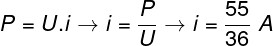Then, as the headlights are connected in series to the same fuse, we must consider that twice the current will pass through this fuse, so: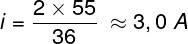Based on the result and the table, the fuse indicated for the function is orange, so the correct alternative is the letter C .

## Solved exercises on electrodynamics

Question 1 — Determine the intensity of the electric current in a wire whose cross section is crossed by 5.10 20 electrons every second. Data: e = 1.6 x 10 -19 C.

a) 60 A

b) 80 A

c) 30A

d) 50 A

Resolution:

Let’s use the formula for electric current that takes into account the quantization of electric charge .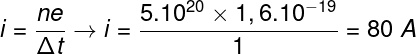Based on our result, the correct alternative is the letter B.

Question 2 — Determine the intensity of the electric current flowing through a conducting wire whose electrical resistance is equal to 0.005 Ω and which is subject to a potential difference of 10V.

a) 2 mA

b) 2 kA

c) 50 mA

d) 0.5 kA

Resolution:

To resolve the issue, it is necessary to use the 1st Ohm’s law.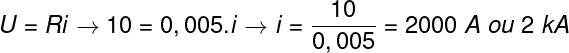Based on the calculation, the correct answer is al etra B.

Question 3 — Determine the electrical power dissipated in the form of heat in a wire with an electrical resistance equal to 0.025 Ω, carried by an electric current of 20.0 A.

a) 10W

b) 0.25 W

c) 0.5 W

d) 5.0 W

Resolution :

To solve the exercise, we must calculate the electrical power dissipated: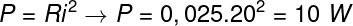Based on the calculation performed, the correct alternative is the letter A .